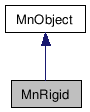# MnRigid Class Reference[OpenMayaFX - API module for effects]

```#include <MnRigid.h>```
Inheritance diagram for MnRigid:[legend]
Collaboration diagram for MnRigid:[legend]

List of all members.

## Detailed Description

Class for wrapping N cloth objects.

This class wraps the internal Maya representation of N cloth objects suitable for use with the Nucleus solver.

## Public Member Functions

MnRigid ()
virtual  ~MnRigid ()
MStatus  setTopology (const int numFaces, int *numVertsPerFace, int *faces, const int numEdges, int *edges)
MStatus  createNRigid ()
MStatus  setPositions (const MFloatPointArray &positions, bool startFrame=true)
MStatus  setVelocities (const MFloatPointArray &velocities)
MStatus  setThickness (float radius)
MStatus  setThickness (float *radius)
MStatus  setBounce (float bounce)
MStatus  setBounce (float *bounce)
MStatus  setFriction (float friction)
MStatus  setFriction (float *friction)
MStatus  setCollisionFlags (const bool vertToVert, const bool edgeToEdge=false, const bool faceToFace=false)
MStatus  getNumVertices (unsigned int &numVerts) const
MStatus  getPositions (MFloatPointArray &positions) const
MStatus  getVelocities (MFloatPointArray &velocities) const
MStatus  getThickness (MFloatArray &radius) const
MStatus  getInverseMass (MFloatArray &inverseMass) const
MStatus  getBounce (MFloatArray &bounce) const
MStatus  getFriction (MFloatArray &friction) const

## Friends

class  MFnNObjectData

## Constructor & Destructor Documentation

 MnRigid::MnRigid ( )

Default constructor. The parent class will set the instance to contain nothing.

 MnRigid::~MnRigid ( ) `[virtual]`

The class destructor.

## Member Function Documentation

 MStatus MnRigid::setTopology ( const int numFaces, int * numVertsPerFace, int * faces, const int numEdges, int * edges )

sets the topology of the underlying N Object. Before calling other methods, this must be the first method you call once the cloth object is created.

Parameters:
 [in] numFaces number of faces [in] numVertsPerFace an array of size numFaces, where each element describes the number of verts on that face [in] faces an array containing the actual face description. Each element is a vertex index. [in] numFaces number of edges [in] edges an array containing the edge description. Each element is a vertex index.
Returns:

 MStatus MnRigid::createNRigid ( )

Creates the underlying Maya TnRigid and sets this class to wrap it.

Returns:

 MStatus MnRigid::setPositions ( const MFloatPointArray & positions, bool startFrame = `true` )

Sets the positions of the vertices of the underlying N cloth object

Parameters:
 [in] positions the array containing the position information [in] startFrame whether this information represents the state of the object at the start frame.
Returns:

 MStatus MnRigid::setVelocities ( const MFloatPointArray & velocities )

Sets the velocities of the vertices of the underlying Ncloth object

Parameters:
 [in] velocities the array containing the velocity information
Returns:

 MStatus MnRigid::setThickness ( float radius )

sets a radius on each point of the mesh for collision purposes. The bigger the radius/thickness, the more easily things collide.

Parameters:
Returns:

 MStatus MnRigid::setThickness ( float * radius )

sets a radius (thickness) on a per point basis for the mesh for collision purposes. The bigger the radius, the more easily things collide.

Parameters:
Returns:

 MStatus MnRigid::setBounce ( float bounce )

sets the bounce for every point in this mesh

Parameters:
 [in] bounce value of bounce to set
Returns:

 MStatus MnRigid::setBounce ( float * bounce )

sets the bounce on a per point basis in this mesh

Parameters:
 [in] bounce value of bounce to set
Returns:

 MStatus MnRigid::setFriction ( float friction )

sets the friction for every point in this mesh

Parameters:
 [in] friction the friction to set
Returns:

 MStatus MnRigid::setFriction ( float * friction )

sets the friction on a per point basis for this mesh

Parameters:
 [in] friction the friction to set
Returns:

 MStatus MnRigid::setCollisionFlags ( const bool vertToVert, const bool edgeToEdge = `false`, const bool faceToFace = `false` )

Sets how (or if) this object will collide with other objects.

Parameters:
 [in] vertToVert whether to calculate vertex - vertex collisions [in] edgeToEdge whether to calculate edge - edge collisions [in] faceToFace whether to calculate face - face collisions
Returns:

 MStatus MnRigid::getNumVertices ( unsigned int & numVerts ) const

Returns the number of vertices in the underlying nRigid

Parameters:
 [out] numVerts Number of vertices.
Returns:

 MStatus MnRigid::getPositions ( MFloatPointArray & positions ) const

gets the positions of the points of the underlying N Object.

Parameters:
 [out] positions the array will be filled with the positions.
Returns:

 MStatus MnRigid::getVelocities ( MFloatPointArray & velocities ) const

gets the velocities of the points of the underlying N cloth object.

Parameters:
 [in] velocities the array will be filled with the positions.
Returns:

 MStatus MnRigid::getThickness ( MFloatArray & radius ) const

gets the thickness at each point of the underlying N rigid object.

Parameters:
 [in] radius the array will be filled with the thickness.
Returns:

 MStatus MnRigid::getInverseMass ( MFloatArray & inverseMass ) const

gets the inverseMass at each point of the underlying N rigid object.

Parameters:
 [in] inverseMass the array will be filled with the inverse masses.
Returns:

 MStatus MnRigid::getBounce ( MFloatArray & bounce ) const

gets the bounce at each point of the underlying N rigid object.

Parameters:
 [in] bounce the array will be filled with the bounce.
Returns:

 MStatus MnRigid::getFriction ( MFloatArray & friction ) const

gets the friction at each point of the underlying N rigid object.

Parameters:
 [in] friction the array will be filled with the friction.
Returns:

 Autodesk® Maya® 2011 © 1997-2010 Autodesk, Inc. All rights reserved. Generated with1.5.6